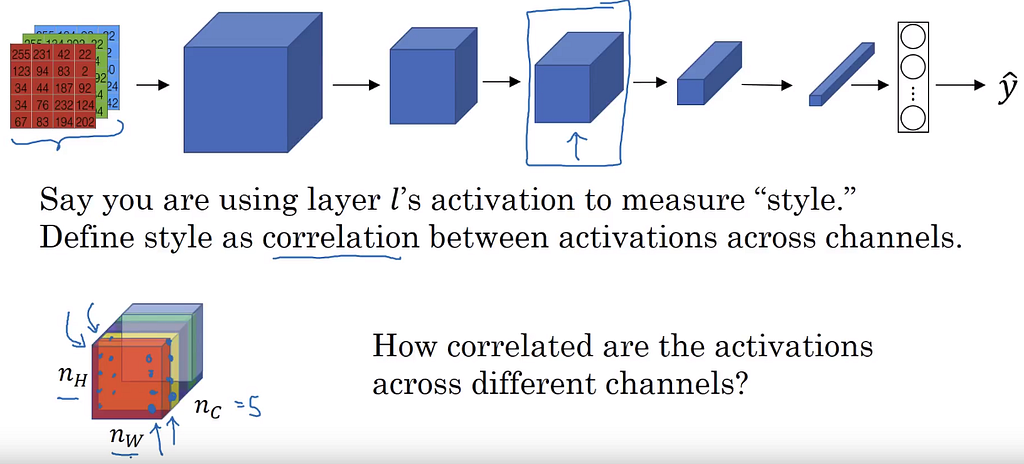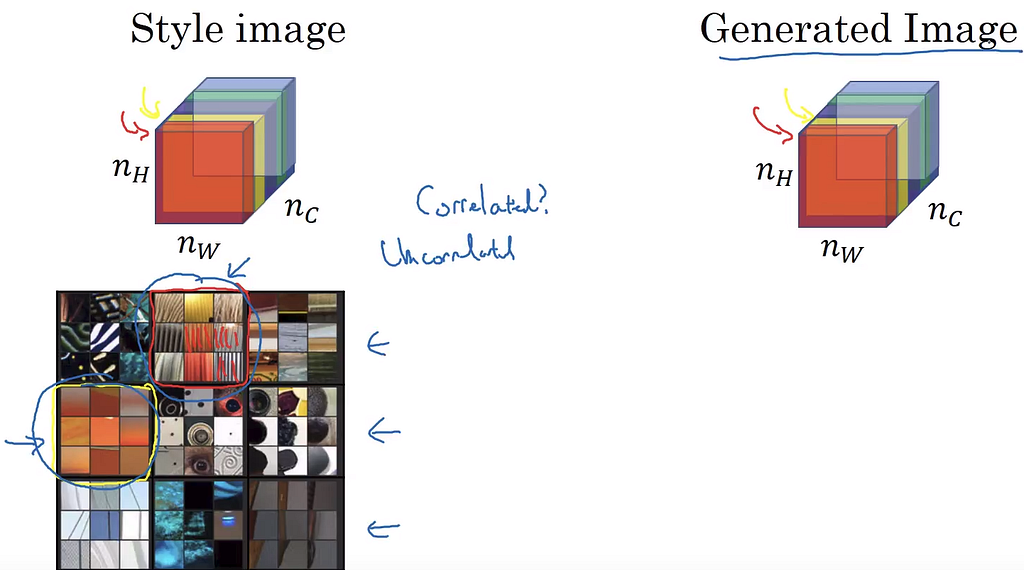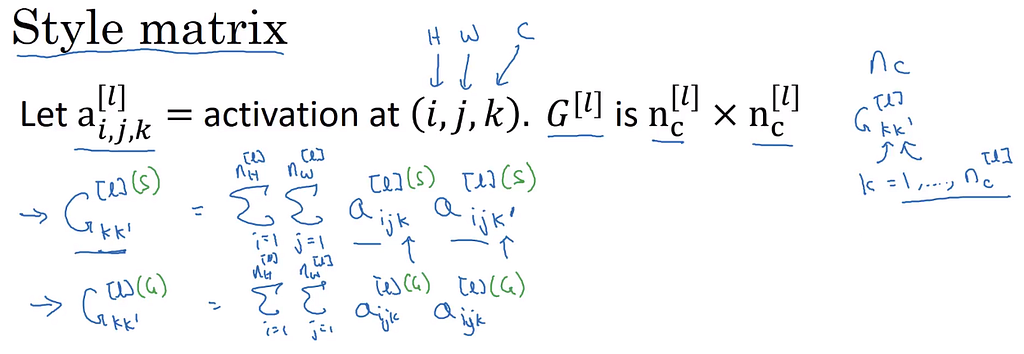# Deeplearning.ai CNN week 4: Special applications

#### One-shot learning

• Learning from one example to recognize the person again.
• Learning a “similarity” function.
• d(img1, img2) = degree of difference between images.
• In certain degree of d value, the verfication works or not.

#### Siamese Network

• Utilize the Convolutional network for transform the input to feature. Doing this by remove the final softmax layer for classification, keep the layer of “128” nodes.
• Each input will be represented by a feature vector after passing this network.
• Then the difference function is defined as: d(x¹, x²) = || f(x¹)- f(x²) ||²_2
• f(x^i) is length of 128

#### Triplet loss

• Look at 3 images in a time.
• Small distance between “anchor” (A) and “positive” (P) image, large distance with the “negative”(N) image.
• But of f(x) is 0, the condition is always satisfy → adding the margin variable (alpha) to keep the equation not return the trivial solution.
• Need the loss as small as possible, or the similarity of A and P plus the margin (alpha) need to be bigger than the similarity of A and N
• Choosing the triplet A, P, N training images is difficult
• Need to choosing the “tough” triplet to train on, to make the gradient descent algorithm to work, otherwise the network weight is no change.
• Typically, companies use a very large face images data for training the Siamese network.

#### Face Verification

• The previous triple loss part for training the representative/ encoding space that can well discriminate images of different people and vice versa.
• The final part is use this encoding to return the final prediction.
• Turn the similarity function to the network based function.
• Adding one final node to return the binary response for two input images is similar or not. Using the logistic regression or chi-square node.
• Face verification problem can be treated as a supervised learning problem.

#### What are deep Convolutional Network learning

• For example in the AlexNet
• Obviously, we can see that the unit in layer 1 is more favorable in “edge” form of image patchs. 9 image patchs of each unit are similar to others in term of color and pattern. Clearly, there are horizontal, vertical, fading, sloping edge in these patchs.
• Follow that, layer 2 contains group of more complex edge patchs or textures. Circle, multiple line pattern ….
• Layer 3 includes much more complex filter, or even clearly some object parts.
• In summary, going to deeper layers, the filters follow this rule: Edge → Textures → more complex image form.

#### Neural style transfer: Cost function

• Three components of the cost function.
• J_content(C,G) how similar the content and generated image
• J_style(S,G) how similar the style and generated image

#### Neural style transfer: Content cost function

• The content here is not pixel-wise difference between two pictures. It is in the Convolution Network content. Noticed that when passing an image to a ConvoNet, it goes through many layers until the end. In each layer, the activation of previous layer input describes how likely/well it fit to a filter.
• Picking the activation information or the response in a certain hidden layer, also describes the “content” information after several kind of “filtering”.

#### Neural style transfer: Style cost function

• What Conv “style” ? the correlation among activation of channels.n_C = 5, visualization of 5 channels activation. Source: deeplearning.ai C4W4L10
• correlation among activation of channels = high level textures co-occur together in a image.The correlation of red/yellow channel of the style image according to the Convnet need to mantain in the generated image. Source: deeplearning.ai C4W4L10
• (i, j, k) height, weight, channel index
• Input style image (s), generated image (G)
• Need to compute all correlation between “pair” of channels to get the overall “style” correlation of an image → store in a matrix G^[l] with l is the lth hidden layer. As n_c channel then G is [n_c x n_c].
• The correlation between “pair” of channels (k,k’) is computing by taking the sum of product of all elements in a channel → return a number.Definition of style matrix of Style image (S) and generated image (G). Source: deeplearning.ai C4W4L10
• The FINAL Style cost function is the Frobenius between two matrices.